PQ Space - Fractals of Mandelbrot type

For documentation of the algorithm, see:
J. A. Shuster, J. Koeplinger, "Doubly nilpotent numbers in the 2D plane" (2010), Appendix A.
http://jenskoeplinger.com/P/PaperShusterKoepl-PQSpace.pdf

License: Creative Commons Share-alike with attribution, v3.0. See the "README" file in the latest package in the Packages directory for details.

Click on the thumbnails below for a 800x800 bitmap, or click on the links under the thumbnails for the command line that created the bitmap, and for the full 5000x5000 pixel bitmap (large).

Last updated: 16 January 2011

(1) PQ Mandelbrot-type fractal in the {p, q} plane, beginning at {[-16, 16], [-16, 16]}, and zooming in to {[-0.5, 0.5], [-0.5, 0.5]}. Radius function r = |cos(2u)|.
Rendered at 5000x5000 pixels, iteration cutoff depth 2000, seed value (0, 0).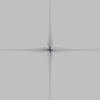full size command Info: p (x-axis) in [-16, 16] q (y-axis) in [-16, 16] Iteration depth: 2000 seed (p, q): (0, 0) Radius r = |cos(2u)| Angle shift: none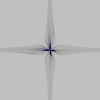full size command Info: p (x-axis) in [-8, 8] q (y-axis) in [-8, 8] Iteration depth: 2000 seed (p, q): (0, 0) Radius r = |cos(2u)| Angle shift: none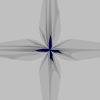full size command Info: p (x-axis) in [-4, 4] q (y-axis) in [-4, 4] Iteration depth: 2000 seed (p, q): (0, 0) Radius r = |cos(2u)| Angle shift: nonefull size command Info: p (x-axis) in [-2, 2] q (y-axis) in [-2, 2] Iteration depth: 2000 seed (p, q): (0, 0) Radius r = |cos(2u)| Angle shift: none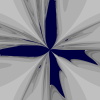full size command Info: p (x-axis) in [-1, 1] q (y-axis) in [-1, 1] Iteration depth: 2000 seed (p, q): (0, 0) Radius r = |cos(2u)| Angle shift: none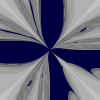full size command Info: p (x-axis) in [-0.5, 0.5] q (y-axis) in [-0.5, 0.5] Iteration depth: 2000 seed (p, q): (0, 0) Radius r = |cos(2u)| Angle shift: none
(2) Select zooms into (1) from above.
Deepest zooms are rendered at iteration depth 5000.full size command Info: p (x-axis) in [0, 0.5] q (y-axis) in [0, 0.5] Iteration depth: 2000 seed (p, q): (0, 0) Radius r = |cos(2u)| Angle shift: none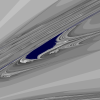full size command Info: p (x-axis) in [0.25, 0.45] q (y-axis) in [0.1, 0.3] Iteration depth: 5000 seed (p, q): (0, 0) Radius r = |cos(2u)| Angle shift: none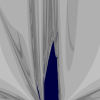full size command Info: p (x-axis) in [-0.8, 0.8] q (y-axis) in [0.3, 1.9] Iteration depth: 2000 seed (p, q): (0, 0) Radius r = |cos(2u)| Angle shift: none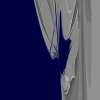full size command Info: p (x-axis) in [0.05, 0.15] q (y-axis) in [0.94, 1.03] Iteration depth: 5000 seed (p, q): (0, 0) Radius r = |cos(2u)| Angle shift: none

(3) PQ Mandelbrot-type fractal with radius function r = cos(2u) i.e. without absolute value choice as in (1) and (2).
Rendered at 5000x5000 pixels, iteration cutoff depth 2000, seed value (0, 0).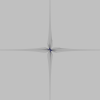full size command Info: p (x-axis) in [-16, 16] q (y-axis) in [-16, 16] Iteration depth: 2000 seed (p, q): (0, 0) Radius r = cos(2u) Angle shift: none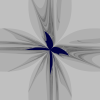full size command Info: p (x-axis) in [-2, 2] q (y-axis) in [-2, 2] Iteration depth: 2000 seed (p, q): (0, 0) Radius r = cos(2u) Angle shift: none

(4) PQ Mandelbrot-type fractal as in (1), i.e. radius function r = |cos(2u)|, but now with iteration seed value varied: ({-1, 0, 1}, {-1, 0, 1})
The following 8 fractals are arranged to correspond to the variation of the seed value.full size command Info: p (x-axis) in [-2, 2] q (y-axis) in [-2, 2] Iteration depth: 2000 seed (p, q): (-1, 1) Radius r = abs(cos(2u)) Angle shift: none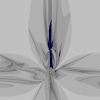full size command Info: p (x-axis) in [-2, 2] q (y-axis) in [-2, 2] Iteration depth: 2000 seed (p, q): (0, 1) Radius r = abs(cos(2u)) Angle shift: none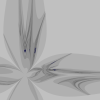full size command Info: p (x-axis) in [-2, 2] q (y-axis) in [-2, 2] Iteration depth: 2000 seed (p, q): (1, 1) Radius r = abs(cos(2u)) Angle shift: none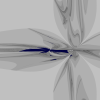full size command Info: p (x-axis) in [-2, 2] q (y-axis) in [-2, 2] Iteration depth: 2000 seed (p, q): (-1, 0) Radius r = abs(cos(2u)) Angle shift: nonefull size command Info: p (x-axis) in [-2, 2] q (y-axis) in [-2, 2] Iteration depth: 2000 seed (p, q): (0, 0) Radius r = abs(cos(2u)) Angle shift: none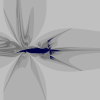full size command Info: p (x-axis) in [-2, 2] q (y-axis) in [-2, 2] Iteration depth: 2000 seed (p, q): (1, 0) Radius r = abs(cos(2u)) Angle shift: none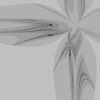full size command Info: p (x-axis) in [-2, 2] q (y-axis) in [-2, 2] Iteration depth: 2000 seed (p, q): (-1, -1) Radius r = abs(cos(2u)) Angle shift: nonefull size command Info: p (x-axis) in [-2, 2] q (y-axis) in [-2, 2] Iteration depth: 2000 seed (p, q): (0, -1) Radius r = abs(cos(2u)) Angle shift: none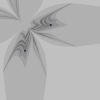full size command Info: p (x-axis) in [-2, 2] q (y-axis) in [-2, 2] Iteration depth: 2000 seed (p, q): (1, -1) Radius r = abs(cos(2u)) Angle shift: none

(5) Select zooms, r = |cos(2u)|, seed value (0, -1)
This is the fractal from (4), bottom row, middle bitmap.full size command Info: p (x-axis) in [-0.6, 0.55] q (y-axis) in [-0.6, 1.6] Iteration depth: 3000 seed (p, q): (0, -1) Radius r = abs(cos(2u)) Angle shift: nonefull size command Info: p (x-axis) in [-0.4, 0.4] q (y-axis) in [-0.4, 0.4] Iteration depth: 4000 seed (p, q): (0, -1) Radius r = abs(cos(2u)) Angle shift: nonefull size command Info: p (x-axis) in [-0.125, -0.07] q (y-axis) in [-0.0275, 0.0275] Iteration depth: 4000 seed (p, q): (0, -1) Radius r = abs(cos(2u)) Angle shift: nonefull size command Info: p (x-axis) in [-0.025, 0.025] q (y-axis) in [-0.025, 0.025] Iteration depth: 4000 seed (p, q): (0, -1) Radius r = abs(cos(2u)) Angle shift: none

(6) Select zooms, r = |cos(2u)|, seed value (-1, 0)
This is the fractal from (4), middle row, left bitmap.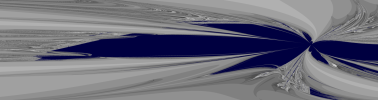full size command Info: p (x-axis) in [-1.4, 0.3] q (y-axis) in [-0.25, 0.2] Iteration depth: 3000 seed (p, q): (-1, 0) Radius r = abs(cos(2u)) Angle shift: nonefull size command Info: p (x-axis) in [-0.11, 0.11] q (y-axis) in [-0.11, 0.11] Iteration depth: 5000 seed (p, q): (-1, 0) Radius r = abs(cos(2u)) Angle shift: none

(7) PQ Mandelbrot-type fractal as in (3), i.e. radius function r = cos(2u), but now with iteration seed value varied: ({-1, 0, 1}, {-1, 0, 1})
The following 8 fractals are arranged to correspond to the variation of the seed value.full size command Info: p (x-axis) in [-2, 2] q (y-axis) in [-2, 2] Iteration depth: 2000 seed (p, q): (-1, 1) Radius r = cos(2u) Angle shift: none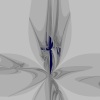full size command Info: p (x-axis) in [-2, 2] q (y-axis) in [-2, 2] Iteration depth: 2000 seed (p, q): (0, 1) Radius r = cos(2u) Angle shift: none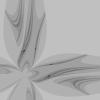full size command Info: p (x-axis) in [-2, 2] q (y-axis) in [-2, 2] Iteration depth: 2000 seed (p, q): (1, 1) Radius r = cos(2u) Angle shift: none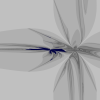full size command Info: p (x-axis) in [-2, 2] q (y-axis) in [-2, 2] Iteration depth: 2000 seed (p, q): (-1, 0) Radius r = cos(2u) Angle shift: nonefull size command Info: p (x-axis) in [-2, 2] q (y-axis) in [-2, 2] Iteration depth: 2000 seed (p, q): (0, 0) Radius r = cos(2u) Angle shift: none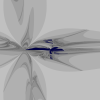full size command Info: p (x-axis) in [-2, 2] q (y-axis) in [-2, 2] Iteration depth: 2000 seed (p, q): (1, 0) Radius r = cos(2u) Angle shift: nonefull size command Info: p (x-axis) in [-2, 2] q (y-axis) in [-2, 2] Iteration depth: 2000 seed (p, q): (-1, -1) Radius r = cos(2u) Angle shift: nonefull size command Info: p (x-axis) in [-2, 2] q (y-axis) in [-2, 2] Iteration depth: 2000 seed (p, q): (0, -1) Radius r = cos(2u) Angle shift: none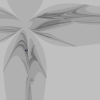full size command Info: p (x-axis) in [-2, 2] q (y-axis) in [-2, 2] Iteration depth: 2000 seed (p, q): (1, -1) Radius r = cos(2u) Angle shift: none

(8) Select zooms, r = cos(2u), with seed values (-1, 0) and (0, 1) respectively
These are the fractals from (7), middle row, left bitmap; and top row middle bitmap.full size command Info: p (x-axis) in [-0.25, 0.25] q (y-axis) in [-0.25, 0.25] Iteration depth: 4000 seed (p, q): (-1, 0) Radius r = cos(2u) Angle shift: nonefull size command Info: p (x-axis) in [-0.5, 0.5] q (y-axis) in [-0.5, 0.5] Iteration depth: 4000 seed (p, q): (0, 1) Radius r = cos(2u) Angle shift: none